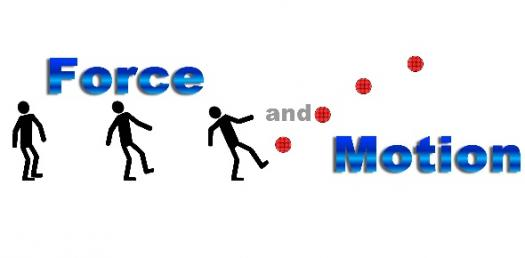# Force, And Motion Test Review

12 Questions | Total Attempts: 2848SettingsCovers force and motion, calculating speed, distance vs time graphs, and speed vs time graphs.

• 1.
If we are not moving then there are no forces acting on us.
• A.

True

• B.

False

• 2.
Friction is a force.
• A.

True

• B.

False

• 3.
The formula for speed is distance divided by time.
• A.

True

• B.

False

• 4.
If an object is not moving then the forces on it are unbalanced.
• A.

True

• B.

False

• 5.
If an object has a net force of 0 then it is not moving.
• A.

True

• B.

False

• 6.
According to this distance vs time graph the object is
• A.

Stopped

• B.

Decelerating

• C.

Moving at a constant speed

• 7.
According to this graph what is the object doing between points A and B?
• A.

Stopped

• B.

Accelerating

• C.

Decelerating

• D.

Constant speed

• 8.
Between what two points is the object accerating?
• A.

0-A

• B.

A-B

• C.

B-C

• D.

This graph does not show the object accelerating.

• 9.
Where on this graph is the object stopped?
• A.

0-A

• B.

A-B

• C.

B-C

• D.

This graph does not show the object stopped.

• 10.
If the man is pulling with a force of 100 Newtons to the left and the elephant made of elements is pulling with a force of 1000 Newtons to the right then what is the net force?
• A.

1100 N left

• B.

900 N left

• C.

1100 N right

• D.

900 N right

• 11.
Ms. Smith left her house at 7:30.  She made the ten mile trip in 30 minutes.  What was her average speed?
• A.

15 mph

• B.

20 mph

• C.

25 mph

• D.

30 mph

• 12.
Force is measured in __________ using a __________.
• A.

Grams, triple beam balance

• B.

Newtons, triple beam balance

• C.

Newtons, spring scale

• D.

Grams, spring scale

Related TopicsBack to top